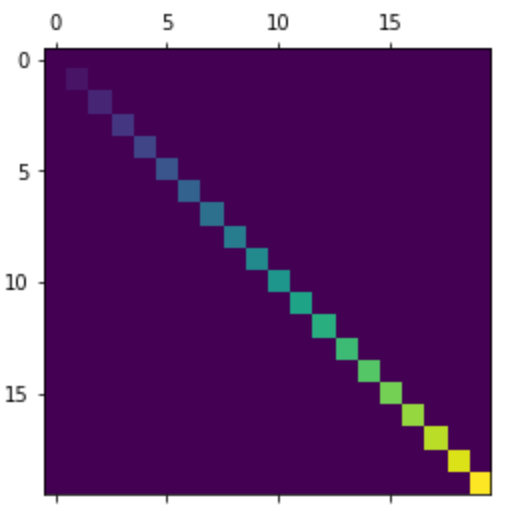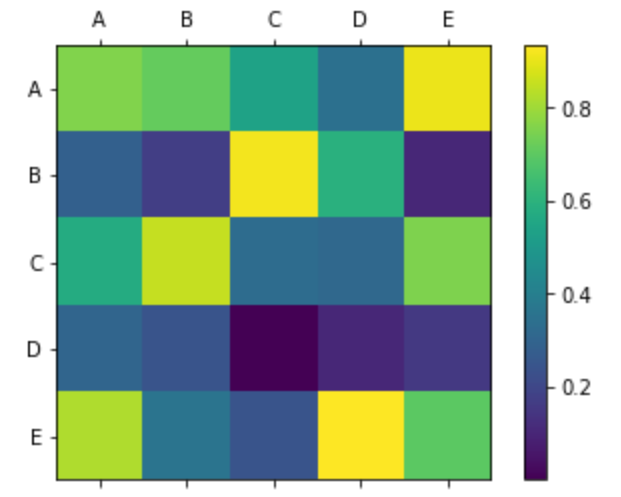# Matplotlib.pyplot.matshow() in Python

Matplotlib is an amazing visualization library in Python for 2D plots of arrays. Matplotlib is a multi-platform data visualization library built on NumPy arrays and designed to work with the broader SciPy stack. It was introduced by John Hunter in the year 2002.

## matplotlib.pyplot.matshow()

matplotlib.pyplot.matshow() function is used to represent an array as a matrix in a new figure window. the upper left-hand corner is set as the origin and the rows (first dimension of the array) are displayed in a horizontal form. The aspect ratio of the figure window is set according to the array to avoid short and narrow figures. The x-axis Tick labels are placed at the top.

Syntax: matplotlib.pyplot.matshow(A, fignum=None, **kwargs)

Parameters:

1. A:: It is an array like object that represents the matrix. It is a required parameter.
2. fignum: It accepts three values namely ‘None’, ‘False’ or an integer value. If the value is set to None it would create a new window of the figure with automated numbering. If the value is a non-zero integer then it is drawn into the figure respective to the given number or it creates it if it doesn’t exist. If the ‘0’ is set as the value of this parameter then it uses the current axes or it creates one if it doesn’t exist.

Returns: It returns an image of Axesimage class.

Other parameters: It aslo accepts the imshow argument for showing image.

Example 1:

 `import` `matplotlib.pyplot as plot ` `import` `numpy as np ` ` `  `# an array with linearly increasing values ` `array ``=` `np.diag(``range``(``20``)) ` ` `  `plot.matshow(array) ` ` `  `plot.show() `

Output:Example 2:

 `import` `numpy as np ` `import` `matplotlib.pyplot as plt ` ` `  `alphabets ``=` `[``'A'``, ``'B'``, ``'C'``, ``'D'``, ``'E'``] ` ` `  `# randomly generated array ` `random_array ``=` `np.random.random((``5``, ``5``)) ` ` `  `figure ``=` `plt.figure() ` `axes ``=` `figure.add_subplot(``111``) ` ` `  `# using the matshow() function  ` `caxes ``=` `axes.matshow(random_array, interpolation ``=``'nearest'``) ` `figure.colorbar(caxes) ` ` `  `axes.set_xticklabels(['']``+``alphabets) ` `axes.set_yticklabels(['']``+``alphabets) ` ` `  `plt.show() `

Output:My Personal Notes arrow_drop_upCheck out this Author's contributed articles.

If you like GeeksforGeeks and would like to contribute, you can also write an article using contribute.geeksforgeeks.org or mail your article to contribute@geeksforgeeks.org. See your article appearing on the GeeksforGeeks main page and help other Geeks.

Please Improve this article if you find anything incorrect by clicking on the "Improve Article" button below.

Article Tags :

Be the First to upvote.

Please write to us at contribute@geeksforgeeks.org to report any issue with the above content.